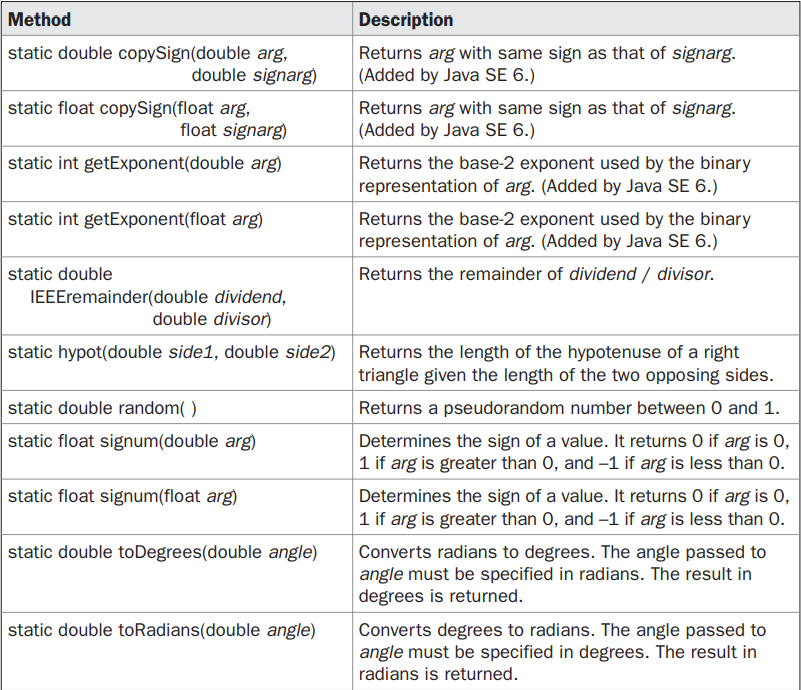Java Math Method

# Java Math Method

Math Methods In Java

Now we will see all the methods in Math in Java
The following methods accept a double parameter for an angle in radians and return the result of their respective transcendental function -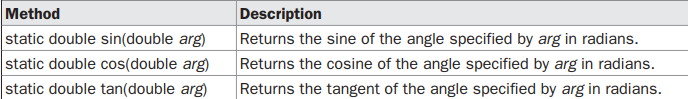The next methods take as a parameter the result of a transcendental function and return, in radians, the angle that would produce that result. They are the inverse of their non-arc companions-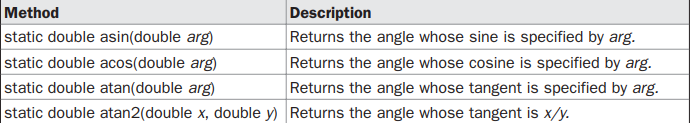The next methods compute the hyperbolic sine, cosine, and tangent of an angle.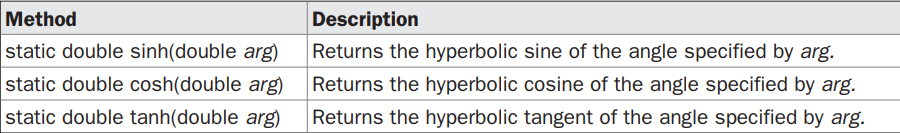Math defines the following exponential methods-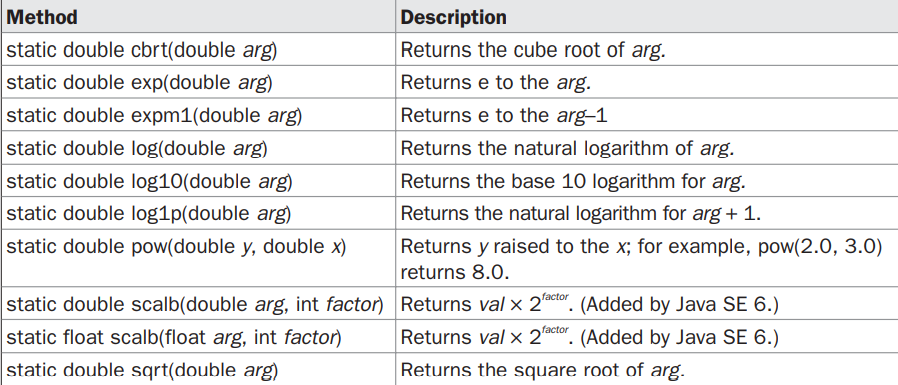The Math class defines several methods that provide various types of rounding operations.Notice the two ulp( ) methods at the end of the table. In this context, ulp stands for units in the last place. It indicates the number of units between a value and the next higher value. It can be used to help assess the accuracy of a result.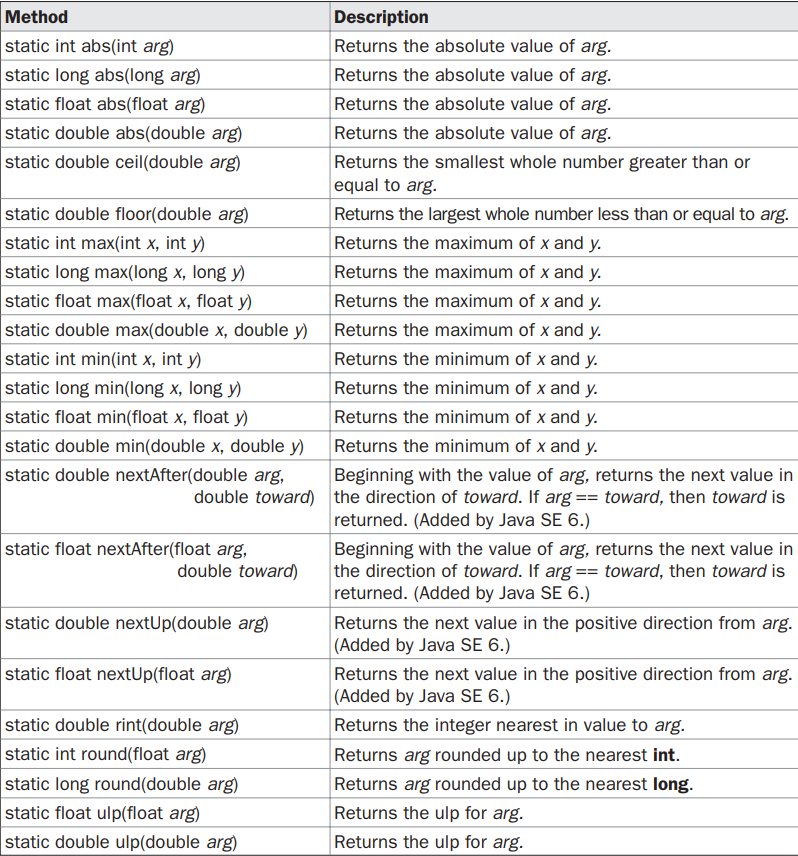In addition to the methods just shown, Math defines several other methods, which are shown here -